Theory and Modern Applications

# Fractional Odd-Dimensional Mechanics

## Abstract

The classical Nambu mechanics is generalized to involve fractional derivatives using two different methods. The first method is based on the definition of fractional exterior derivative and the second one is based on extending the standard velocities to the fractional ones. Fractional Nambu mechanics may be used for nonintegrable systems with memory. Further, Lagrangian which is generate fractional Nambu equations is defined.

## 1. Introduction

Derivatives and integrals of fractional-order have found many applications in recent studies in mechanics and physics, for example, in chaotic dynamics, quantum mechanics, plasma physics, anomalous diffusion, and so many fields of physics . Fractional mechanics describes both conservative and nonconservative systems [13, 14]. In mechanics, Riewe has shown that Lagrangian involving fractional time derivatives leads to equation of motion with nonconservative classical derivatives such as friction [13, 14]. Motivated by this approach many researchers have explored this area giving new insight into this problem . Agrawal has presented fractional Euler-Lagrangian equation involving Riemann-Liouville derivatives [16, 17]. Further fractional single and multi-time Hamiltonian formulation has been developed by Baleanu and coworkers .

In 1973, Nambu generalized Hamiltonian mechanics which is called now Nambu mechanics. This formalism is shown that provide a suitable framework for the odd dimensional phase space and nonintegrable systems . By this motivation the authors have fractionalized this formalism .

In this work two methods are introduced for fractionalizing of Nambu mechanics. The first method is based on the definition of fractional exterior derivative and fractional forms. The second methods is based on fractionalizing of classical velocity. The resulted equations using these methods may use for complex memorial systems.

This paper is organized as follows.

Section 2 is devoted to a brief review of the fractional derivative definitions and fractional forms. Section 3 contains the classical Nambu mechanics. Section 4 deals with fractionalizing Hamiltonian mechanics using fractional differential forms. Using two different methods in Section 5 the Nambu mechanics has been fractionalized. In Section 6 is defined a Lagrangian which its variation gives the fractional Hamiltonian equations. In Section 7 we present our conclusions.

## 2. Basic Tools

The following subsections contain all mathematical tools used in this manuscript.

### 2.1. Fractional Derivatives

In this section it is briefly presented the definition of the left and the right fractional derivatives of Riemann-Liouville as well as Caputo [6, 7]. The left Riemann-Liouville fractional derivative is defined as(2.1)

and the right Riemann-Liouville fractional derivative,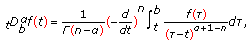(2.2)

where the orderfulfillsandrepresent the gamma function. An alternative definition of Riemann-Liouville fractional derivative called Caputo derivative that introduced by Caputo in 1967. The left Caputo derivative defined as(2.3)

and the right Caputo fractional derivative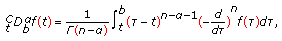(2.4)

where the ordersatisfies. The Riemann-Liouville derivative of constant isn't zero, but the Caputo derivative of a constant is zero.

### 2.2. Fractional Forms

The calculus of classical differential forms is a powerful tool in applied mathematics. There are so many books that give a clear introduction to this field . In calculus when a new function appears in the scene, it is natural to ask what its derivative is. Similarly with form, it is reasonable to ask what its exterior derivative is. For example a 1-form, integer-order one, can be shown as follows: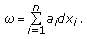(2.5)

The classical exterior derivative is defined as(2.6)

In [45, 46] the author generalizes the definition of integer-order vector spaces form to fractional-order one, and denotes it by. In this notationis the order of differential form,the number of coordinate differential appearing in the basis elements,the number of coordinates. For instance (2.5) is an element of.

The Definition of Fractional Exterior Derivative

If the partial derivative in the definition of the classical exterior derivative, is replaced by the fractional-order, definition of fractional exterior derivative is obtained,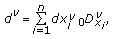(2.7)

whereis left fractional derivative. Let,(2.8)

and consider its fractional exterior derivative(2.9)

Using the product rule of exterior fractional derivative,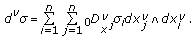(2.10)

If, then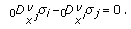(2.11)

Note that in the following equations, thesign is omitted between the differential forms.

## 3. Nambu Mechanics

Consider the Hamilton equations(3.1)

In another notation(3.2)

Nambu generalized these equations to tripletdynamical variables with two Hamiltoniansas follows: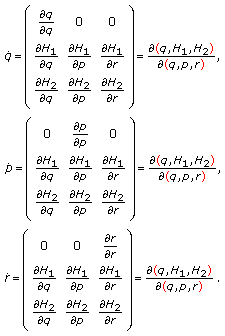(3.3)

Considering these equations in terms of differential from, take the following 1-form:(3.4)

The exterior derivative ofis(3.5)

The Pfaffian equations is obtained, and then the Hamiltonian equations are resulted.

Now in terms of differential forms the Nambu mechanics is obtained using the following form: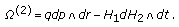(3.6)

The exterior derivative of this form is as follows: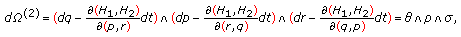(3.7)

where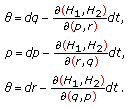(3.8)

Now the Paffian equations. Equating them with zero we lead to Nambu mechanics equations.

## 4. Fractional Hamilton's Equations

The fractional generalization of (3.4) can be defined by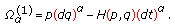(4.1)

The fractional exterior derivative of this form is as follows:(4.2)

Taking(4.3)

we have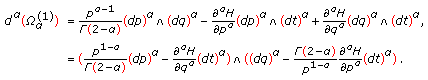(4.4)

The fractional Pfaffian equations are as follows: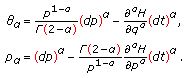(4.5)

The fractional Hamiltonian equations is resulted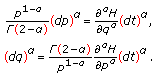(4.6)

## 5. Fractional Nambu Mechanics

Method 1.

In this part, we present the fractional Nambu mechanic based on fractional generalization of the form (3.6). As a result, we obtain(5.1)

Taking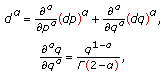(5.2)

the exterior of this form is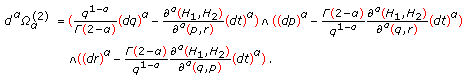(5.3)(5.4)

It can be generalized as follows: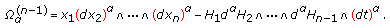(5.5)

Method 2.

In this part we present the fractional Nambu mechanics based on two Lagrangian formulation. In simple fractional Hamiltonian equations we have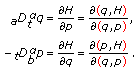(5.6)

If we generalize these equations to Nambu fractional: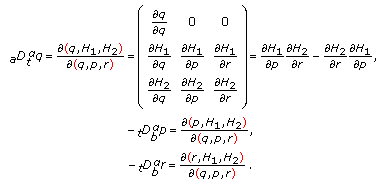(5.7)

In classical mechanics only variable,, is considered as a configuration variables and variables,are first and second canonical variables, respectively. Suppose that it could be existed as many Lagrangian as the number of Hamiltonian. In the simplest case suppose that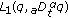and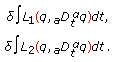(5.8)

Then the Fractional Euler-Lagrangian equations are(5.9)

Next are defined the first and second canonical momentum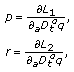(5.10)

and then the fractional Euler-Lagrange equations are(5.11)

Now we define two Hamiltoniansandas follows: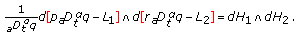(5.12)

Therefore we obtain(5.13)

By expanding the right hand and by comparing the coefficients of form we lead to fractional Nambu mechanic equations.

This result can be generalized to the-dimensional case, namely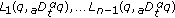. As before we calculate the momenta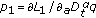,...,, and so on(5.14)

## 6. A Fractional Lagrangian Formulation

Let us assumed that the functionsare of two variable. Then the corresponding Lagrangian is given by the following form: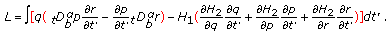(6.1)

Imposing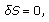(6.2)

we obtain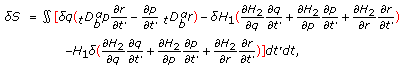(6.3)

or(6.4)

After some calculations, we obtain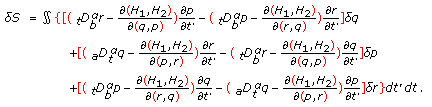(6.5)

We conclude that if (5.7) is satisfied, thenfor arbitrary variations ofand.

## 7. Conclusions

In this paper, we defined new equations corresponding to the complex systems described by the Nambu mechanics within the languages of the fractional differential forms. It is shown that variation of the corresponding new action using fractional Lagrangian gives fractional Nambu equations. The equivalent methods presented in this manuscript can be applied to investigate the dynamics of the complex nonintegrable systems with memory. The classical results are obtained in the limiting case.

## References

1. Sabatier J, Agrawal OP, Tenreiro Machado JA: Advance in Fractional Calculus: Theoretical Developments and Applications in Physics and Engineering. Springer, Dordrecht, The Netherlands; 2007:xiv+552.

2. Baleanu D, Guvenc Ziya B, Tenreiro MJA: New Trends in Nanotechnology and Fractional Calculus Applications. Springer, Dordrecht, The Netherlands; 2009.

3. Hilfer R: Applications of Fractional Calculus in Physics. World Scientific, Singapore; 2000:viii+463.

4. Gorenflo R, Mainardi F: Fractional Calculus: Integral and Differential Equations of Fractional Orders, Fractals and Fractional Calculus in Continuum Mechanics. Springer, New York, NY, USA; 1997.

5. Kilbas AA, Srivastava HM, Trujillo JJ: Theory and Applications of Fractional Differential Equations, North-Holland Mathematics Studies. Volume 204. Elsevier, Amsterdam, The Netherlands; 2006:xvi+523.

6. Oldham KB, Spanier J: The Fractional Calculus. Academic Press, New York, NY, USA; 1974:xiii+234.

7. Miller KS, Ross B: An Introduction to the Fractional Integrals and Derivatives-Theory and Application, A Wiley-Interscience Publication. John Wiley & Sons, New York, NY, USA; 1993:xvi+366.

8. Podlubny I: Fractional Differential Equations, Mathematics in Science and Engineering. Volume 198. Academic Press, San Diego, Calif, USA; 1999:xxiv+340.

9. Magin RL: Fractional Calculus in Bioengineering. Begell House, Connecticut, Mass, USA; 2006.

10. Samko SG, Kilbas AA, Marichev OI: Fractional Integrals and Derivatives. Gordon and Breach, New York, NY, USA; 1993:xxxvi+976.

11. West BJ, Bologna M, Grigolini P: Physics of Fractal Operators, Institute for Nonlinear Science. Springer, New York, NY, USA; 2003:x+354.

12. Zaslavsky GM: Hamiltonian Chaos and Fractional Dynamics. Oxford University Press, Oxford, UK; 2005:xiv+421.

13. Riewe F: Nonconservative Lagrangian and Hamiltonian mechanics. Physical Review E 1996,53(2):1890-1899. 10.1103/PhysRevE.53.1890

14. Riewe F: Mechanics with fractional derivatives. Physical Review E 1997,55(3):3581-3592. 10.1103/PhysRevE.55.3581

15. Golmankhaneh AK, Golmankhaneh AK, Baleanu D, Baleanu MC: Hamiltonian structure of fractional first order Lagrangian. International Journal of Theoretical Physics 2010,49(2):365-375. 10.1007/s10773-009-0209-5

16. Agrawal OP: Formulation of Euler-Lagrange equations for fractional variational problems. Journal of Mathematical Analysis and Applications 2002,272(1):368-379. 10.1016/S0022-247X(02)00180-4

17. Agrawal OP: Fractional variational calculus and the transversality conditions. Journal of Physics A 2006,39(33):10375-10384. 10.1088/0305-4470/39/33/008

18. Baleanu D, Muslih SI: Lagrangian formulation of classical fields within Riemann-Liouville fractional derivatives. Physica Scripta 2005,72(2-3):119-121. 10.1238/Physica.Regular.072a00119

19. Baleanu D, Agrawal OP: Fractional Hamilton formalism within Caputo's derivative. Czechoslovak Journal of Physics 2006,56(10-11):1087-1092. 10.1007/s10582-006-0406-x

20. Baleanu D, Muslih SI, Taş K: Fractional Hamiltonian analysis of higher order derivatives systems. Journal of Mathematical Physics 2006,47(10):8.

21. Baleanu D, Golmankhaneh AK, Golmankhaneh AK: Fractional Nambu mechanics. International Journal of Theoretical Physics 2009,48(4):1044-1052. 10.1007/s10773-008-9877-9

22. Frederico GSF, Torres DFM: A formulation of Noether's theorem for fractional problems of the calculus of variations. Journal of Mathematical Analysis and Applications 2007,334(2):834-846. 10.1016/j.jmaa.2007.01.013

23. Golmankhaneh AK: Fractional poisson bracket. Turkish Journal of Physics 2008,32(5):241-250.

24. Klimek M: Fractional sequential mechanics—models with symmetric fractional derivative. Czechoslovak Journal of Physics 2001,51(12):1348-1354. 10.1023/A:1013378221617

25. Klimek M: Lagrangean and Hamiltonian fractional sequential mechanics. Czechoslovak Journal of Physics 2002,52(11):1247-1253. 10.1023/A:1021389004982

26. Laskin N: Fractional quantum mechanics. Physical Review E 2000,62(3 A):3135-3145.

27. Laskin N: Fractional Schrödinger equation. Physical Review E 2002,66(5):-7.

28. Muslih SI, Baleanu D: Hamiltonian formulation of systems with linear velocities within Riemann-Liouville fractional derivatives. Journal of Mathematical Analysis and Applications 2005,304(2):599-606. 10.1016/j.jmaa.2004.09.043

29. Rabei EM, Nawafleh KI, Hijjawi RS, Muslih SI, Baleanu D: The Hamilton formalism with fractional derivatives. Journal of Mathematical Analysis and Applications 2007,327(2):891-897. 10.1016/j.jmaa.2006.04.076

30. Rabei EM, Tarawneh DM, Muslih SI, Baleanu D: Heisenberg's equations of motion with fractional derivatives. Journal of Vibration and Control 2007,13(9-10):1239-1247. 10.1177/1077546307077469

31. Rabei EM, Almayteh I, Muslih SI, Baleanu D: Hamilton-Jacobi formulation of systems within Caputo's fractional derivative. Physica Scripta 2008.,77(1):

32. Tarasov VE: Fractional variations for dynamical systems: Hamilton and Lagrange approaches. Journal of Physics A 2006,39(26):8409-8425. 10.1088/0305-4470/39/26/009

33. Tarasov VE: Fractional generalization of gradient and Hamiltonian systems. Journal of Physics A 2005,38(26):5929-5943. 10.1088/0305-4470/38/26/007

34. Tarasov VE: Fractional vector calculus and fractional Maxwell's equations. Annals of Physics 2008,323(11):2756-2778. 10.1016/j.aop.2008.04.005

35. Zaslavsky GM: Chaos, fractional kinetics, and anomalous transport. Physics Reports A 2002,371(6):461-580. 10.1016/S0370-1573(02)00331-9

36. Baleanu D, Golmankhaneh AK, Nigmatullin R, Golmankhaneh AK: Fractional Newtonian mechanics. Central European Journal of Physics 2010,8(1):120-125. 10.2478/s11534-009-0085-x

37. Golmankahneh AK, Golmankahneh AK, Baleanu D: On nonlinear fractional Klein Gordab equation. Signal Processing 2010,91(3):466-451.

38. Baleanu D, Golmankhaneh AK, Golmankhaneh AK: The dual action of fractional multi time Hamilton equations. International Journal of Theoretical Physics 2009,48(9):2558-2569. 10.1007/s10773-009-0042-x

39. Nambu Y: Generalized Hamiltonian dynamics. Physical Review D 1973, 7: 2405-2412. 10.1103/PhysRevD.7.2405

40. Mukunda N, Sudarshan ECG: Relation between Nambu and Hamiltonian mechanics. Physical Review D 1976,13(10):2846-2850. 10.1103/PhysRevD.13.2846

41. Takhtajan L: On foundation of the generalized Nambu mechanics. Communications in Mathematical Physics 1994,160(2):295-315. 10.1007/BF02103278

42. Fecko M: On geometrical formulation of the Nambu dynamics. Journal of Mathematical Physics 1992,33(3):926-929. 10.1063/1.529744

43. Cayley A: Collected Mathematical Papers. Cambridge University Press, Cambridge, UK; 1890.

44. Flanders H: Differential Forms with Applicatons to the Physics Sciences, Dover Books on Advanced Mathematics. 2nd edition. Dover, New York, NY, USA; 1989:xvi+205.

45. Shepherd C, Naber M: Fractional differential forms II. http://arxiv.org/abs/math-ph/0301016

46. Cottrill-Shepherd K, Naber M: Fractional differential forms. Journal of Mathematical Physics 2001,42(5):2203-2212. 10.1063/1.1364688

## Author information

Authors

### Corresponding author

Correspondence to Dumitru Baleanu.

## Rights and permissions

Reprints and Permissions

Khalili Golmankhaneh, A., Golmankhaneh, A., Baleanu, D. et al. Fractional Odd-Dimensional Mechanics. Adv Differ Equ 2011, 526472 (2011). https://doi.org/10.1155/2011/526472

• Accepted:

• Published:

• DOI: https://doi.org/10.1155/2011/526472

### Keywords

• Fractional Derivative
• Exterior Derivative
• Fractional Form
• Hamiltonian Mechanic
• Dimensional Phase Space